Balancing Car - Part 2

1. Set up the interrupt/timer

The 1-core CPU of the Arduino Board is expected to operate at two modes at the same time - receive the data from the MPU board through I2C and manage the PID controller. Both of the two tasks are being exeuted in realtime so the only way to manage this is using an interrupt.

The strategy here is to trigger the interrupt service routine (ISR) every 20 us to deliver the PID results at THAT moment to the stepper motors. Compare to the mechanical movement of the motor, the 20 us activation is nothing so it will be able to adjust the movement accordingly and smoothly.

Use the following code to set up the ISR in your setup() function:

TWBR = 12;                                                                //Set the I2C clock speed to 400kHz

//To create a variable pulse for controlling the stepper motors a timer is created that will execute a piece of code (subroutine) every 20us

//This subroutine is called TIMER2_COMPA_vect
TCCR2A = 0;                                                               //Make sure that the TCCR2A register is set to zero
TCCR2B = 0;                                                               //Make sure that the TCCR2A register is set to zero
TIMSK2 |= (1 << OCIE2A);                                                  //Set the interupt enable bit OCIE2A in the TIMSK2 register
TCCR2B |= (1 << CS21);                                                    //Set the CS21 bit in the TCCRB register to set the prescaler to 8
OCR2A = 39;                                                               //The compare register is set to 39 => 20us / (1s / (16.000.000MHz / 8)) - 1
TCCR2A |= (1 << WGM21);                                                   //Set counter 2 to CTC (clear timer on compare) mode

Now let's take a look at the function of the registers in the datasheet.

On Page 292, the TWBR register is explained:The 328p is being driven by a 16 MHz crystal, if the factor is set at 12. The equation to calculate the bit rate is on Page 267: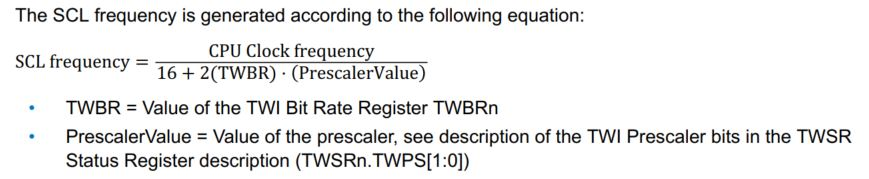The CPU clock frequency is 16M Hz, TWBR=12, PrescalerValue = 1. The PrescalerValue is determined by the TWSR register, on Page 293: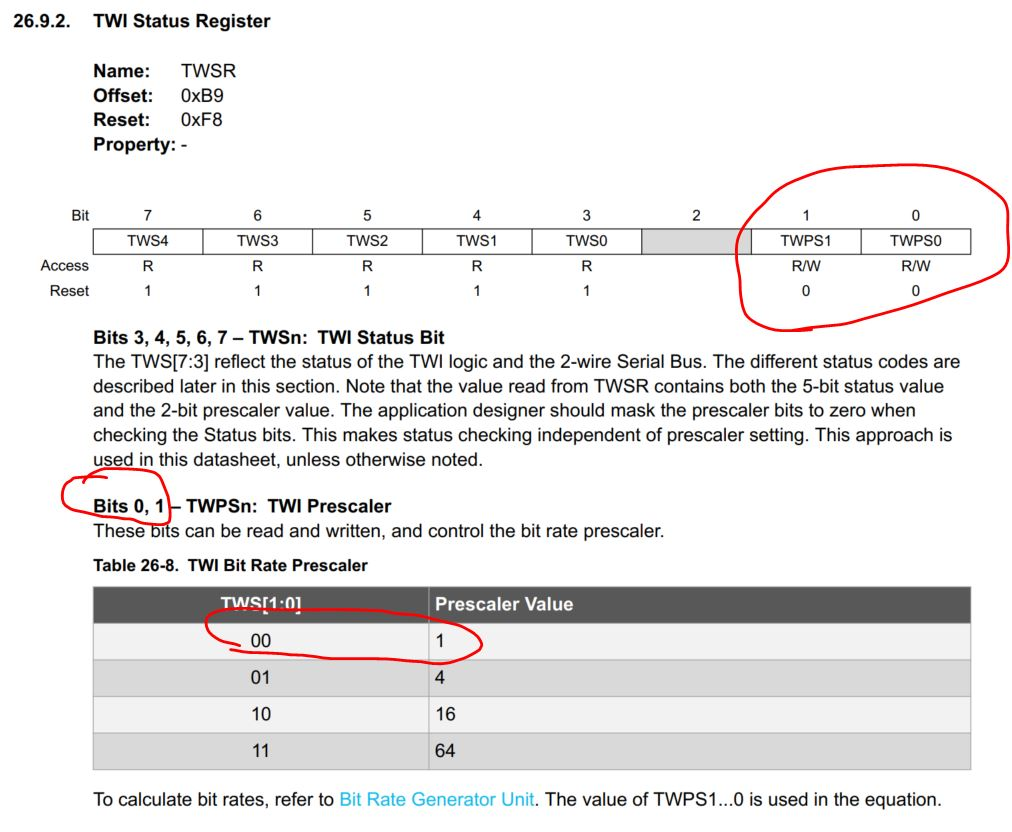The default values are 00 for bit 1:0, so the corresponding PrescalerValue is 1.

So the bit rate for the I2C communication (SCL) is 16M/(16+2x12)=400 kHz.

The TIMSK2 register: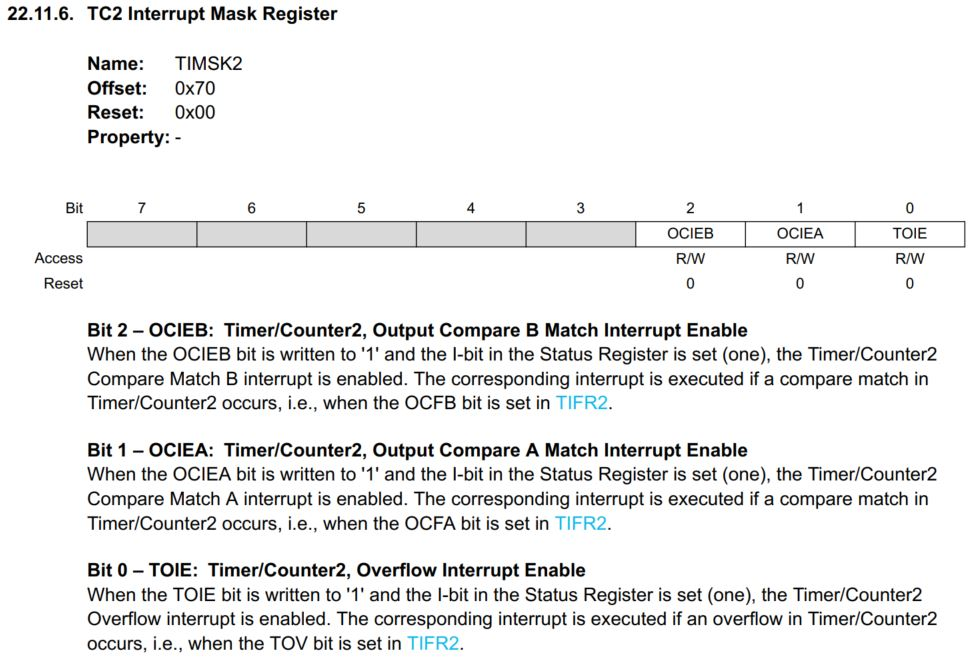As the OCIEA (or OCIE2A) is set to 1, the Compare Match A Interrupt mode is enabled.

The TCCR2B register, set the prescaler to 8: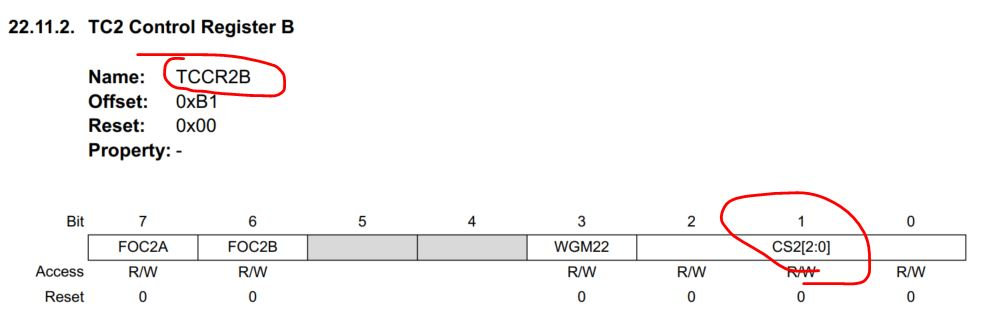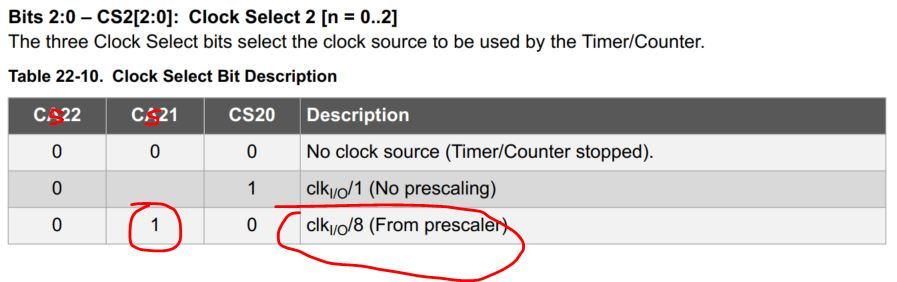Set the OCR2A register, which stores the number to compare, to 39: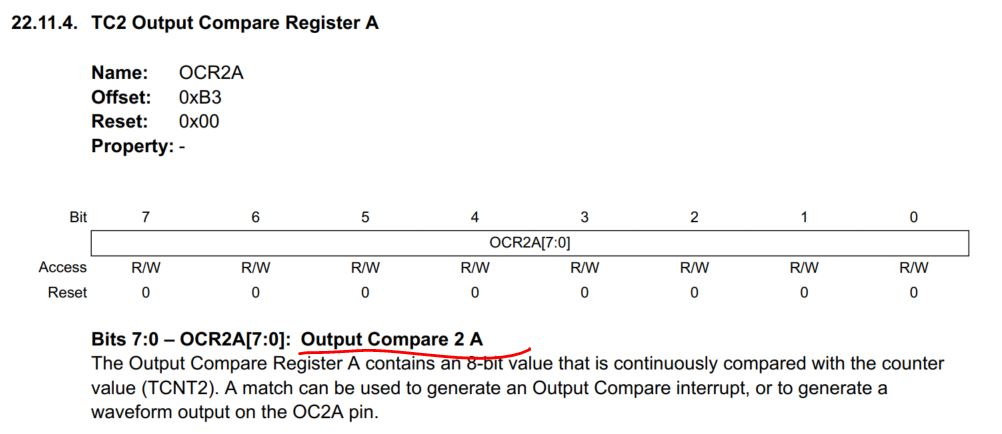In that case, the ISR will be triggered for every 16M/8Toggle on each match: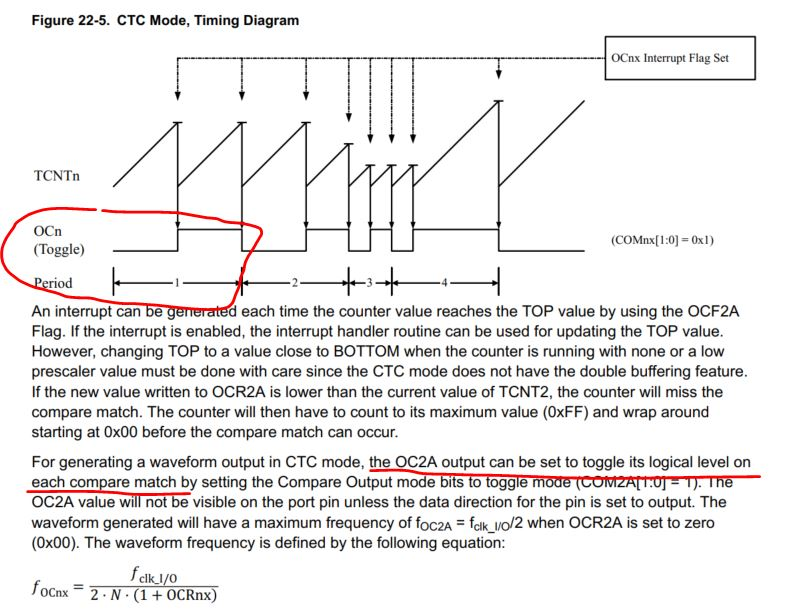Which means every 20 us it toggles and trigger the ISR, so the period is actually 40 us, so the equation becomes:

1/40us = 16M/(2x8x(1+x)), and x = 39

Finally, set it at the CTC mode: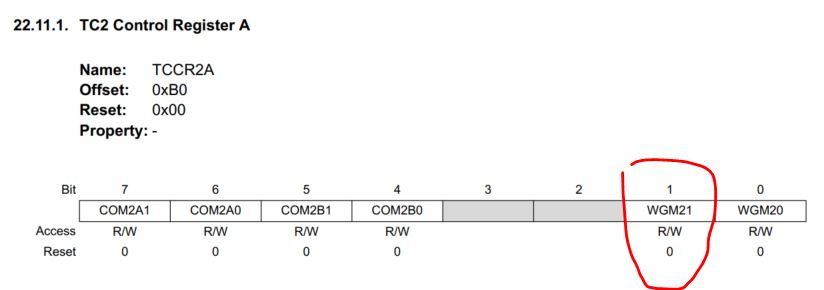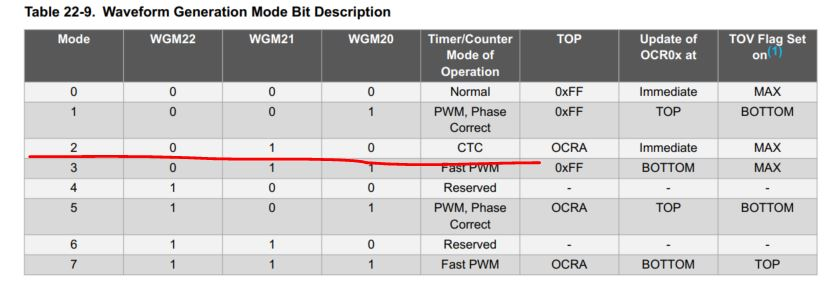2. The demonstration sketch to get you started

The following sketch contains the entire 'loop()' function that limits the accelerometer data within the -8192 and 8192 range. The 8192 was predefined as explained in the previous tutorial:

The binary value occasionally overflows and goes above 8192 slightly. Use a IF Statement to limit it within the range.Read the following sketch. You can use it for your first test. It can be directly used as the loop() function of the your sketch. It'll let the car stand still.

void loop(){
Wire.beginTransmission(MPU);                                              //Start communication with the gyro
Wire.write(0x3F);                                                         //Start reading at register 3F
Wire.endTransmission();                                                   //End the transmission
Wire.requestFrom(MPU, 2);                                                 //Request 2 bytes from the gyro
accelerometer_data_raw += acc_calibration_value;                          //Add the accelerometer calibration value
if(accelerometer_data_raw > 8192)accelerometer_data_raw = 8192;           //Limiting the acc data to +/-8192;
if(accelerometer_data_raw < -8192)accelerometer_data_raw = -8192;
angle_acc = asin((float)accelerometer_data_raw/8192.0)* 57.296;           //Calculate the current angle according to the accelerometer
Wire.beginTransmission(MPU);                                              //Start communication with the gyro
Wire.write(0x45);                                                         //Start reading at register 45
Wire.endTransmission();                                                   //End the transmission
Wire.requestFrom(MPU, 2);                                                 //Request 2 bytes from the gyro
gyro_pitch_data_raw -= gyro_pitch_calibration_value;                      //Add the gyro calibration value
angle_gyro += gyro_pitch_data_raw * 0.000031;                             //Calculate the traveled during this loop angle and add this to the angle_gyro variable
angle_gyro = angle_gyro * 0.9996 + angle_acc * 0.0004;                    //Correct the drift of the gyro angle with the accelerometer angle
pid_error_temp = angle_gyro-0;                                            //Find the error between the current angle and 0

pid_i_mem += pid_i_gain * pid_error_temp;                                 //Calculate the I-controller value and add it to the pid_i_mem variable
if(pid_i_mem > 400)pid_i_mem = 400;                                       //Limit the I-controller to the maximum controller output
else if(pid_i_mem < -400)pid_i_mem = -400;
//Calculate the PID output value
pid_output = pid_p_gain * pid_error_temp + pid_i_mem + pid_d_gain * (pid_error_temp - pid_last_d_error);
if(pid_output > 400)pid_output = 400;                                     //Limit the PI-controller to the maximum controller output
else if(pid_output < -400)pid_output = -400;
pid_last_d_error = pid_error_temp;                                        //Store the error for the next loop

if(pid_output < 5 && pid_output > -5)pid_output = 0;                      //Create a dead-band to stop the motors when the robot is balanced
if(pid_output==0){ // if the car is balanced, do not output any power to the motor
delayToBeAdded=0; // if delayToBeAdded is 0, in the ISR, it will never trigger the motor.
}
else {
delayToBeAdded = 5500/(abs(pid_output) + 9); // to linearize the output
}
//The angle calculations are tuned for a loop time of 4 milliseconds. To make sure every loop is exactly 4 milliseconds a wait loop
//is created by setting the loop_timer variable to +4000 microseconds every loop.
while(loop_timer > micros()); // while loop_timer > micros(), hold there and wait
loop_timer += 4000;           // once loop_timer < micros(), add 4000 us to loop_timer.
}

The ISR function will be invoked every 20 us. Every time it enters it, the counter_motor variable will increase by 1, then it is immediately compared to the 'delayToBeAdded' variable. If 'delaytoBeAdded' is large (a small pid_out value), it takes many 20 us cycles to satisfy the condition of the IF Satement, then clear the counter_motor variable. Otherwise, the ISR will basically do nothing except for the increment of counter_motor variable.

After counter_motor is reset to 0 in ISR, the 'else if' conditions will be satisfied the next time it enters the ISR and add A step to the wheels.

ISR(TIMER2_COMPA_vect){
counter_motor ++;
if (counter_motor > delayToBeAdded){ // this if statement block only defines the direction of the two motors.
counter_motor=0;
if (pid_output>0){
PORTD &= 0b00000000; //0b00DSDS00 (D: Dir, S: Step), reset all bits for Port D is just preparing for the line below it.
PORTD |= 0b00001000; //Two different directions for the two steppers on two sides. (the car leans forward)
}
else {
PORTD &= 0b00000000;
PORTD |= 0b00100000; // Two different directions for the two steppers on two sides. (the car leans backward)
}
}
// The following lines will provide a step to the stepper motor
else if(counter_motor == 1) PORTD |= 0b00010100;             //Set output 2 high to create a pulse for the stepper controller
else if(counter_motor == 2) PORTD &= 0b11101011;             //Set output 2 low because the pulse only has to last for 20us
}

A demonstration video:

This is the end of this tutorial. The tasks for you is to refer to the tutorial of the YABR website and build a wirelessly controlled 2-wheel balanced car. You can choose to use your smartphone or a joystick to control it. Make a PCB and a 3D printing case for you product.

Reference:
1. The original YABR balancing robot. part 1, part 2, part 3
2. A duplicate by mikerobotscience.
.
4. A balanced stick.
5. The Cubli: a cube that can jump up, balance, and 'walk'.
6. Drones that join together and fly.